Skip to main content

# Expected Value of Exponential Fuzzy Number and Its Application to Multi-item Deterministic Inventory Model for Deteriorating Items

## Abstract

Possibility, necessity, and credibility measures play a significant role to measure the chances of occurrence of fuzzy events. In this paper, possibility, necessity, and credibility measures of exponential fuzzy number, and its expected value has been derived. A multi-item two-warehouse deterministic inventory model for deteriorating items with stock-dependent demand has been developed. For the proposed inventory model, the different costs and other parameters are considered in exponential fuzzy nature. Solution methodology of this model using expected value has been discussed. A numerical example is considered to illustrate the multi-item two-warehouse deterministic inventory model. Finally, few sensitivity analyses are presented under different rates of deterioration to check the validity of the proposed model.

## Introduction

Commonly speaking, uncertainty is usual to all real-life problems, for example fuzziness and randomness. Since Zadeh  introduced the fuzzy set theory, it has been well developed and applied in a wide variety of real-life problems. Possibility theory was proposed by Zadeh  and developed by many researchers, e.g., Dubois and Prade , Klir , Yager  and others. A self dual measure called credibility measure was introduced by Liu and Liu . The mean value of a fuzzy number was introduced by Dubois and Prade . Thereafter, Carlsson and Fuller  defined a possiblistic mean and variance of fuzzy numbers. The expected value of fuzzy variable using possibility theory was proposed by Hilpern , and application of expected value operator called expected value model was introduced by Liu and Liu .

Classical economic order quantity model which was developed in 1965 had the specific requirement of deterministic cost and demand. Classical inventory models generally deal with a single item. But in real-world situations, a single-item inventory rarely occurs and multi-item inventory is common. Ghare and Schrader  were the first researchers to develop an economic order quantity model (EOQ) for an item with exponential decay. A multi-item inventory model with constant demand and infinite replenishment under the restriction on total average shortage, shortage area, and total average inventory investment cost developed by Das et al. . Maiti and Maiti  investigated a production policy for damageable items with variable cost function in an imperfect production process, and Mondal and Maiti  developed a multi-item EOQ model. Recently, many researchers studied on multi-item EOQ inventory model such as Mousavi et al. , Pasandideh et al. , Wee et al. , Lau and Lau , Nahmias and Schmidt , and Vairaktarakis .

In general, deterioration in the inventory system is defined as damage, decay, spoilage, evaporation, pilferage, obsolescence, etc.. The classical economic order quantity (EOQ) inventory model developed in 1915 had the specific requirements of deterministic cost and lack of deterioration of the stock item. A finite rate of production with a variable rate of deterioration inventory model was formulated by Misra . Raafat  was the first researcher to develop a continuous deterioration of the on-hand inventory model and later more discussion by Goyel and Giri . From then on, the inventory models of deteriorating items in different manners were developed by many researchers such as Benkherouf , Cohen , Kang and Kim , Goyal and Gunasekaran .

The deterministic inventory model with two levels of shortage and infinite replenishment rates developed by Sarma  and this model extended by Murdeshwar and Sathe . Pakkala and Achary  improved a deterministic inventory model for deteriorating items with two warehouses and finite replenishment rate. Bhunia and Maiti  proposed a two-warehouse inventory model for deteriorating items with a linear trend in demand and shortages. Goswami and Chaudhuri  first introduced the inventory model with two storage and stock-dependent demand rates. A multi-warehouse inventory model for multi-items with time-varying demand and shortage was developed by Zhou .

In many cases, the parameters in inventory problems may not be crisp and be somewhat vague in nature. For example, the holding cost for an item is supposed to be dependent on the amount of storage. Similarly, the replenishment cost depends upon the total quantity to be produced in a scheduling period. Moreover, because of the inventory system, the total profit in a scheduling period may be uncertain, and uncertainties may be associated with these variables and the above goals and parameters are normally vague and imprecise, i.e., fuzzy in nature. Maximum total average profit is imprecise in a practical inventory problem. In these situations, fuzzy set theory can be used for the formulation of inventory models. Maity and Maiti  solved a multi-item fuzzy inventory model with possibility and necessity constraints. Yao and Lee  developed an inventory model considering fuzzy order quantity, fuzzy production quantity, and fuzzy demand. Nia et al.  developed a multi-item EOQ model under shortage with fuzzy vendor managed. Recently, many researchers such as Kar et al.  and Roy and Maiti  have developed several fuzzy inventory models.

In spite of the abovementioned developments, following additions can also be made in exponential fuzzy number, the formulation and solution of inventory models for deteriorating items under imprecise environments.

• Possibility, necessity, and credibility measures of exponential trapezoidal fuzzy number

• Expected value of exponential trapezoidal fuzzy number based on credibility measure

• Multi-item two-warehouse deterministic inventory model for deteriorating items with stock dependent demand

• Expected value method to solve a multi-item inventory model for deteriorating items

The rest of the paper is organized as follows: In Section “Basic Preliminaries”, we present some basic knowledge of exponential trapezoidal fuzzy number and its arithmetic operations. Section “Possibility Necessity and Credibility Measures of Exponential Trapezoidal Fuzzy Number” provides the possibility, necessity, and credibility measures of exponential trapezoidal fuzzy number and its graphical representation. In Section “Expected Value of Exponential Trapezoidal Fuzzy Number”, expected value of exponential trapezoidal fuzzy number has been discussed. Section“Assumption and Notations” shows the assumptions and notations for the mathematical model. In Section“Mathematical Formulation”, mathematical formulations are derived and the solution procedure is discussed. Numerical examples are given in Section“Numerical Illustration” to validate the proposed method. In Section “Sensitivity Analysis”, sensitivity analysis is made for the change of deterioration and is depicted using figures. Finally, conclusion and the scope of future research come in Section “Conclusion”.

## Basic Preliminaries

### Definition 1

Exponential trapezoidal fuzzy number(ETFN): [ 38 ] Let $$\tilde {a}=\left (a_{1},a_{2},a_{3},a_{4}\right) \left (a_{i} \in \mathbb {R}, i=1,2,3,4 \right.$$ and a 1a 2a 3a 4) be a ETFN, then its membership function (cf. Fig. 1) defined as follows

$$\mu_{\tilde{a}}(x)=\left\{ \begin{array}{ll} \exp \left\{-\frac{(a_{2}-x)}{(a_{2}-a_{1})}\right\}, & \quad \text{if } a_{1}\leq x \leq a_{2}\\ 1, & \quad \text{if } a_{2} \leq x \leq a_{3}\\ \exp \left\{-\frac{(x-a_{3})}{(a_{4}-a_{3})}\right\}, & \quad \text{if } a_{3}\leq x \leq a_{4} \end{array} \right.$$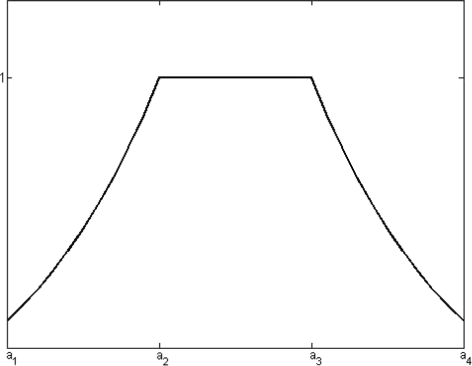Fig. 1

### Definition 2

Let $$\tilde {a}=\left (a_{1},a_{2},a_{3},a_{3},a_{4}\right)$$ and $$\tilde {b}=\left (b_{1},b_{2},b_{3},b_{4}\right)$$ be two ETFN, then

1. (i)

$$\tilde {a}\oplus \tilde {b}=\{a_{1}+b_{1}, a_{2}+b_{2}, a_{3}+b_{3}, a_{4}+b_{4}\}$$

2. (ii)

$$\tilde {a}\ominus \tilde {b}=\{a_{1}-b_{4}, a_{2}-b_{3}, a_{3}-b_{2}, a_{4}-b_{1}\}$$

3. (iii)

$$\tilde {a}\otimes \tilde {b}\approx \{a_{1}b_{1}, a_{2}b_{2}, a_{3}b_{3}, a_{4}b_{4}\}$$

4. (iv)

$$k\tilde {a}=\left \{ \begin {array}{ll} \left (ka_{1},ka_{2},ka_{3},ka_{4}\right) & \quad \text {if } k \geq 0\\ \left (ka_{4},ka_{3},ka_{2},ka_{1}\right) & \quad \text {if } k<0 \end {array}\right.$$

### Definition 3

Let $$\tilde {a}=\left (a_{1},a_{2},a_{3},a_{4}\right)$$ and $$\tilde {b}=\left (b_{1},b_{2},b_{3},b_{4}\right)$$ be two ETFN with membership function $$\mu _{\tilde {a}}$$, $$\mu _{\tilde {b}}$$ respectively, and let $$\mathbb {R}$$ be the set of real numbers. Then

$$Pos\left(\tilde{a} {\odot} {\tilde{b}}\right)=\sup \left\{\mu_{\tilde{a}}(x)\wedge\mu_{\tilde{b}}(y):x,y\in\mathbb{R}; x \odot y \right\}$$

and

$$Nec\left({\tilde{a}} {\odot} {\tilde{b}}\right)=\inf \left\{\mu_{\tilde{a}}(x)\vee\mu_{\tilde{b}}(y):x,y\in\mathbb{R}; x \odot y \right\}$$

where Pos represents the possibility of membership function and Nec represents the necessity of membership function, denote any relation of =,>,<,≤,≥ and =max,=min respectively.

### Definition 4

let $$\tilde {a}=\left (a_{1},a_{2},a_{3},a_{4}\right)$$ be a ETFN. Then the measure of $$\tilde {a}$$ defined as

$$Me(\tilde{a})=\lambda Pos(\tilde{a})+(1-{\lambda})Nec(\tilde{a})$$

where λ(0≤λ≤1) is the optimistic-pessimistic parameter, we determine the combined nature of decision maker λ, as follows If λ=1, then Me=Pos; it means the decision maker is optimistic and maximum chance of $$\tilde {a}$$ holds. If λ=0, then Me=Nec; it means the decision maker is pessimistic and minimal chance of $$\tilde {a}$$ holds. If λ=0.5, then Me=Cr; it means the decision maker takes compromise attitude of $$\tilde {a}$$ holds. where Cr is the credibility measure and defined by $$Cr=\frac {Pos+Nec}{2}$$.

## Possibility Necessity and Credibility Measures of Exponential Trapezoidal Fuzzy Number

Let $$\tilde {a}=\left (a_{1},a_{2},a_{3},a_{4}\right)$$ and $$\tilde {b}=\left (b_{1},b_{2},b_{3},b_{4}\right)$$ be two ETFN. From Definition 3, possibility of $$\tilde {a} \leq \tilde {b}$$ and $$\tilde {a} \geq \tilde {b}$$ are defined as follows

$$\begin{array}{@{}rcl@{}} Pos\left(\tilde{a}\leq \tilde{b}\right)=\left\{ \begin{array}{ll} 1, & \quad \text{if}~ a_{2} \leq b_{3}\\ \exp \left\{-\frac{(a_{2}-b_{3})}{(b_{4}-b_{3})+(a_{2}-a_{1})}\right\}, & \quad \text{if }~ a_{2}>b_{3}, b_{4}>a_{1}\\ \end{array}\right. \end{array}$$
(1)

and

$$\begin{array}{@{}rcl@{}} Pos\left(\tilde{a}\geq \tilde{b}\right)=\left\{ \begin{array}{ll} 1, & \quad \text{if } ~b_{2} \leq a_{3}\\ \exp \left\{-\frac{(b_{2}-a_{3})}{(a_{4}-a_{3})+(b_{2}-b_{1})}\right\}, & \quad \text{if } ~b_{2}>a_{3}, a_{4}>b_{1}\\ \end{array}\right. \end{array}$$
(2)

In particular the possibility measure of $$\tilde {a} \leq x$$(cf. Fig. 2) and $$\tilde {a} \geq x$$(cf. Fig. 3) are

$$\begin{array}{@{}rcl@{}} Pos\left(\tilde{a}\leq x\right)=\left\{ \begin{array}{ll} 1, & \quad \text{if } ~x \geq a_{2}\\ \exp \left\{-\frac{(a_{2}-x)}{(a_{2}-a_{1})}\right\}, & \quad \text{if ~} a_{1} \leq x \leq a_{2}\\ \end{array}\right. \end{array}$$
(3)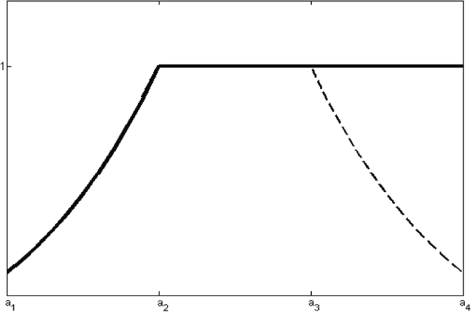Fig. 2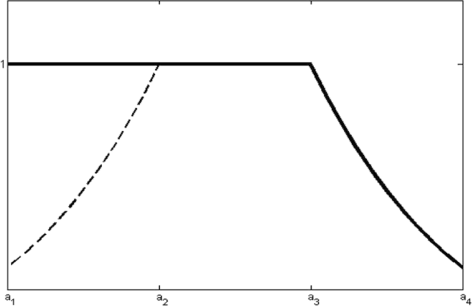Fig. 3

and

$$\begin{array}{@{}rcl@{}} Pos\left(\tilde{a}\geq x\right)=\left\{ \begin{array}{ll} 1, & \quad \text{if } x \leq a_{3}\\ \exp \left\{ -\frac{(x-a_{3})}{(a_{4}-a_{3})}\right\}, & \quad \text{if } a_{3} \leq x \leq a_{4}\\ \end{array}\right. \end{array}$$
(4)

Now from Definition 3, we evaluated necessity measure of $$\tilde {a} \leq \tilde {b}$$ and $$\tilde {a} \geq \tilde {b}$$ are as follows

$$\begin{array}{@{}rcl@{}} Nec\left(\tilde{a}\leq \tilde{b}\right)=\left\{ \begin{array}{ll} 0, & \quad \text{if } b_{1} \leq a_{3}\\ 1-\exp \left\{ -\frac{(a_{3}-b_{1})}{(b_{2}-b_{1})+(a_{3}-a_{4})}\right\}, & \quad \text{if } b_{1}>a_{3}, a_{4}>b_{2}\\ \end{array}\right. \end{array}$$
(5)

and

$$\begin{array}{@{}rcl@{}} Nec\left(\tilde{a}\geq \tilde{b}\right)=\left\{ \begin{array}{ll} 0, & \quad \text{if } a_{2} \leq b_{4}\\ 1-\exp \left\{ -\frac{(a_{2}-b_{4})}{(a_{2}-a_{1})+(b_{3}-b_{4})}\right\}, & \quad \text{if } a_{2}>b_{4}, a_{1}<b_{3}\\ \end{array}\right. \end{array}$$
(6)

In particular the necessity measure of $$\tilde {a} \leq x$$(cf. Fig. 4) and $$\tilde {a} \geq x$$(cf. Fig. 5) are

$$\begin{array}{@{}rcl@{}} Nec\left(\tilde{a}\leq x\right)=\left\{ \begin{array}{ll} 0, & \quad \text{if } x \leq a_{3}\\ 1-\exp \left\{ -\frac{(a_{3}-x)}{(a_{3}-a_{4})}\right\}, & \quad \text{if } a_{3} \leq x \leq a_{4}\\ \end{array}\right. \end{array}$$
(7)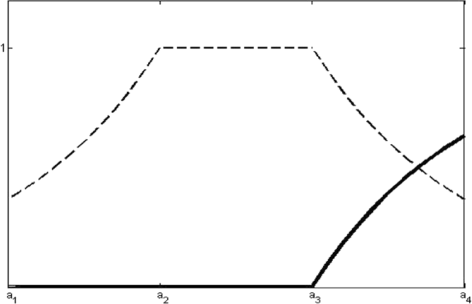Fig. 4Fig. 5

and

$$\begin{array}{@{}rcl@{}} Nec\left(\tilde{a}\geq x\right)=\left\{ \begin{array}{ll} 0, & \quad \text{if } x \geq a_{2}\\ 1-\exp \left\{ -\frac{(x-a_{2})}{(a_{1}-a_{2})}\right\}, & \quad \text{if } a_{1} \leq x \leq a_{2}\\ \end{array}\right. \end{array}$$
(8)

From Definition 4, measure of $$\tilde {a} \leq x$$(cf. Fig. 6) and $$\tilde {a} \geq x$$(cf. Fig. 7) can be defined as follows

$$\begin{array}{@{}rcl@{}} Me\left(\tilde{a}\leq x\right)=\left\{ \begin{array}{ll} \lambda \exp\left\{-\frac{(a_{2}-x)}{(a_{2}-a_{1})} \right \},& \quad \text{if } a_{1}\leq x\leq a_{2}\\ \lambda, & \quad \text{if } a_{2} \leq x \leq a_{3}\\ 1-(1-\lambda)\exp \left\{ -\frac{(x-a_{3})}{(a_{4}-a_{3})}\right\}, & \quad \text{if } a_{3} \leq x \leq a_{4}\\ \end{array}\right. \end{array}$$
(9)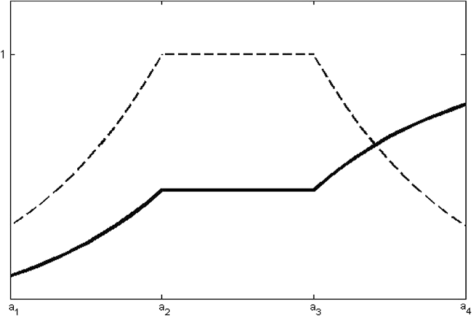Fig. 6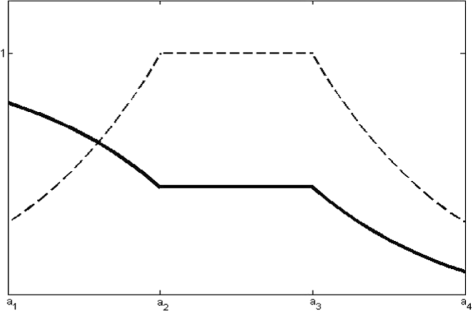Fig. 7

and

$$\begin{array}{@{}rcl@{}} Me\left(\tilde{a}\geq x\right)=\left\{ \begin{array}{ll} 1-(1-\lambda)\exp\left\{-\frac{(a_{2}-x)}{(a_{2}-a_{1})} \right \},& \quad \text{if } a_{1}\leq x\leq a_{2}\\ \lambda, & \quad \text{if } a_{2} \leq x \leq a_{3}\\ \lambda \exp \left\{ -\frac{(x-a_{3})}{(a_{4}-a_{3})}\right\}, & \quad \text{if } a_{3} \leq x \leq a_{4}\\ \end{array}\right. \end{array}$$
(10)

When $$\lambda =\frac {1}{2}$$, measure denoted as credibility measure

$$\begin{array}{@{}rcl@{}} Cr\left(\tilde{a}\leq x\right)=\left\{ \begin{array}{ll} \frac{1}{2} \exp\left\{-\frac{(a_{2}-x)}{(a_{2}-a_{1})} \right \},& \quad \text{if } a_{1}\leq x\leq a_{2}\\ \frac{1}{2}, & \quad \text{if } a_{2} \leq x \leq a_{3}\\ 1-\frac{1}{2}\exp \left\{ -\frac{(x-a_{3})}{(a_{4}-a_{3})}\right\}, & \quad \text{if } a_{3} \leq x \leq a_{4}\\ \end{array}\right. \end{array}$$
(11)

and

$$\begin{array}{@{}rcl@{}} Cr\left(\tilde{a}\geq x\right)=\left\{ \begin{array}{ll} 1-\frac{1}{2} \exp\left\{-\frac{(a_{2}-x)}{(a_{2}-a_{1})} \right \},& \quad \text{if } a_{1}\leq x\leq a_{2}\\ \frac{1}{2}, & \quad \text{if } a_{2} \leq x \leq a_{3}\\ \frac{1}{2}\exp \left\{ -\frac{(x-a_{3})}{(a_{4}-a_{3})}\right\}, & \quad \text{if } a_{3} \leq x \leq a_{4}\\ \end{array}\right. \end{array}$$
(12)

## Expected Value of Exponential Trapezoidal Fuzzy Number

### Definition 5

Let $$\tilde {a}=\left (a_{1},a_{2},a_{3},a_{4}\right)$$ be a ETFN, then the expected value of $$\tilde {a}$$ defined as follows

$$E^{Me}(\tilde{a})=\int_{0}^{\infty}Me\lbrace \tilde{a} \geq x\rbrace dx-\int_{-\infty}^{0}Me\lbrace \tilde{a} \leq x\rbrace dx$$
(13)

### Theorem 1

Let $$\tilde {a}=(a_{1},a_{2},a_{3},a_{4})$$ be a ETFN. Then its expected value is

{{} \begin{aligned} E^{Me}(\tilde{a})=\left\{ \begin{array}{ll} \left(\left(1\,-\,\frac{1}{e}\right)\frac{\lambda}{a_{4}-a_{3}}\,-\,\frac{(1-\lambda)}{a_{2}-a_{1}}\,+\,\frac{\lambda}{a_{2}-a_{1}}\frac{1}{e}\right)\,+\,\exp\left(\!-\frac{a_{2}}{a_{2}-a_{1}}\right) & \quad \!\!\!\!\!\text{if}~ a_{1} \!\leq \!0\! \leq a_{2}\\ \left(\frac{(1-\lambda)}{a_{2}-a_{1}}-\frac{\lambda}{a_{2}-a_{1}}\right)+\lambda(a_{3}-a_{2})+a_{2}, \\ \left(1-\frac{1}{e}\right)\left(\frac{\lambda}{a_{4}-a_{3}}-\frac{\lambda}{a_{2}-a_{1}}\right)+\lambda (a_{3}+a_{2}), & \quad\!\!\!\!\!\! \text{if } a_{2} \!\leq\! 0 \leq a_{3}\\ \left(\frac{(1-\lambda)}{a_{4}-a_{3}}-\frac{\lambda}{a_{4}-a_{3}}\frac{1}{e}-\frac{\lambda}{a_{2}-a_{1}}\left(1-\frac{1}{e}\right)\right)+\exp \left(\frac{a_{3}}{a_{4}-a_{3}}\right) \\ \left(\frac{\lambda}{a_{4}-a_{3}}-\frac{(1-\lambda)}{a_{4}-a_{3}}\right)-\lambda (a_{3}-a_{2})+a_{3}, & \quad\!\!\!\!\!\! \text{if } a_{3} \!\leq\! 0 \leq a_{4} \end{array}\right. \end{aligned}}

### Proof

Since there 3 cases, let’s discuss every case in turn

Case 1: a 1≤0≤a 2

$$\begin{array}{@{}rcl@{}} E^{Me}(\tilde{a})&=&\int_{0}^{\infty}Me\lbrace\tilde{a} \geq x\rbrace dx-\int_{-\infty}^{0}Me\lbrace\tilde{a} \leq x\rbrace dx \\ &=&\int_{0}^{a_{2}}Me\lbrace\tilde{a} \geq x\rbrace dx +\int_{a_{2}}^{a_{3}}Me\lbrace\tilde{a} \geq x\rbrace dx+ \int_{a_{3}}^{a_{4}}Me\lbrace\tilde{a} \geq x\rbrace dx\\ & & +\int_{a_{4}}^{\infty}Me\lbrace\tilde{a} \geq x\rbrace dx -\left(\int_{-\infty}^{a_{1}}Me\lbrace\tilde{a}\leq x\rbrace dx+\int_{a_{1}}^{0}Me\lbrace\tilde{a} \leq x\rbrace dx \right) \\ &=&\int_{0}^{a_{2}} \left(1-(1-\lambda)\exp\left(-\frac{a_{2}-x}{a_{2}-a_{1}}\right)\right)dx+\int_{a_{2}}^{a_{3}}\lambda dx\\ & &+\int_{a_{3}}^{a_{4}}\lambda \exp \left(-\frac{x-a_{3}}{a_{4}-a_{3}}\right)dx-\int_{a_{1}}^{0}\lambda \exp\left(-\frac{a_{2}-x}{a_{2}-a_{1}}\right)dx\\ &=& \left(\left(1-\frac{1}{e}\right)\frac{\lambda}{a_{4}-a_{3}}-\frac{(1-\lambda)}{a_{2}-a_{1}}+\frac{\lambda}{a_{2}-a_{1}}\frac{1}{e}\right)+\exp\left(-\frac{a_{2}}{a_{2}-a_{1}}\right)\\ & & \left(\frac{(1-\lambda)}{a_{2}-a_{1}}-\frac{\lambda}{a_{2}-a_{1}}\right)+\lambda(a_{3}-a_{2})+a_{2}\\ \end{array}$$

Case 2: a 2≤0≤a 3

$$\begin{array}{@{}rcl@{}} E^{Me}(\tilde{a})&=&\int_{0}^{\infty}Me\lbrace\tilde{a} \geq x\rbrace dx-\int_{-\infty}^{0}Me\lbrace \tilde{a} \leq x\rbrace dx \\ &=&\int_{0}^{a_{3}}Me\lbrace \tilde{a} \geq x\rbrace dx +\int_{a_{3}}^{a_{4}}Me\lbrace \tilde{a} \geq x\rbrace dx+ \int_{a_{4}}^{\infty}Me\lbrace \tilde{a} \geq x\rbrace dx\\ & & -\left(\int_{-\infty}^{a_{1}}Me\lbrace \tilde{a} \leq x\rbrace dx +\int_{a_{1}}^{a_{2}}Me\lbrace \tilde{a} \leq x\rbrace dx+\int_{a_{2}}^{0}Me\lbrace \tilde{a} \leq x\rbrace dx\right) \\ &=& \int_{0}^{a_{3}}\lambda dx \,+\, \int_{a_{3}}^{a_{4}} \lambda \exp\left(\!-\frac{x-a_{3}}{a_{4}-a_{3}}\right)dx\,-\,\left(\int_{a_{1}}^{a_{2}}\lambda\exp\left(\!-\frac{a_{2}-x}{a_{2}-a_{1}}\right)dx\,+\,\int_{a_{2}}^{0}\lambda dx\right)\\ &=&\left(1-\frac{1}{e}\right)\left(\frac{\lambda}{a_{4}-a_{3}}-\frac{\lambda}{a_{2}-a_{1}}\right)+\lambda (a_{3}+a_{2}) \end{array}$$

Case 3: a 3≤0≤a 4

$$\begin{array}{@{}rcl@{}} E^{Me}(\tilde{a})&=&\int_{0}^{\infty}Me\lbrace \tilde{a} \geq x\rbrace dx-\int_{-\infty}^{0}Me\lbrace \tilde{a} \leq x\rbrace dx \\ &=&\int_{0}^{a_{4}}Me\lbrace \tilde{a} \geq x\rbrace dx +\int_{a_{4}}^{\infty}Me\lbrace \tilde{a} \geq x\rbrace dx-\left(\int_{-\infty}^{a_{1}}Me\lbrace \tilde{a} \leq x\rbrace dx \right.\\ & & \left. +\int_{a_{1}}^{a_{2}}Me\lbrace \tilde{a} \leq x\rbrace dx +\int_{a_{2}}^{a_{3}} Cr\lbrace \tilde{a} \leq x\rbrace dx+\int_{a_{3}}^{0}Me\lbrace \tilde{a} \leq x\rbrace dx\right) \\ &=&\int_{0}^{a_{4}}\lambda \exp\left(-\frac{x-a_{3}}{a_{4}-a_{3}}\right)dx\\ & & \!\!\!\!\!\!\!-\left(\int_{a_{1}}^{a_{2}}\lambda \exp\left(\!\!-\frac{a_{2}-x}{a_{2}-a_{1}}\right)dx\,+\,\int_{a_{2}}^{a_{3}}\lambda dx\,+\,\int_{a_{3}}^{0}\left(\!1\!-(1\!-\lambda)\exp \left(\!\!-\frac{x-a_{3}}{a_{4}-a_{3}} \right) \right) dx \right) \\ &=&\left(\frac{(1-\lambda)}{a_{4}-a_{3}}-\frac{\lambda}{a_{4}-a_{3}}\frac{1}{e}-\frac{\lambda}{a_{2}-a_{1}}\left(1-\frac{1}{e}\right)\right)+\exp \left(\frac{a_{3}}{a_{4}-a_{3}}\right) \\ & & \left(\frac{\lambda}{a_{4}-a_{3}}-\frac{(1-\lambda)}{a_{4}-a_{3}}\right)-\lambda (a_{3}-a_{2})+a_{3} \end{array}$$

### Remarks 1

When $$\lambda =\frac {1}{2}$$, we have

$$E^{Cr}(\tilde{a})=\frac{1}{2}\left(1-\frac{1}{e}\right)\left(\frac{1}{a_{4}-a_{3}}-\frac{1}{a_{2}-a_{1}}\right)+\frac{1}{2}(a_{2}+a_{3})$$

### Definition 6

Let $$\tilde {a}=\left (a_{1},a_{2},a_{3},a_{4}\right)$$ be a ETFN, then the expected value of $$\tilde {a}$$ is defined by

$$E(\tilde{a})=\frac{1}{2}\left(1-\frac{1}{e}\right)\left(\frac{1}{a_{4}-a_{3}}-\frac{1}{a_{2}-a_{1}}\right)+\frac{1}{2}(a_{2}+a_{3})$$

### Definition 7

If a 2=a 3, i.e, $$\tilde {a}$$ degenerates to exponential triangular fuzzy number(ETrFN), then the expected value of ETrFN $$\tilde {a}$$ is

$$E(\tilde{a})=\frac{1}{2}\left(1-\frac{1}{e}\right)\left(\frac{2a_{2}-a_{1}-a_{4}}{(a_{2}-a_{1})(a_{4}-a_{2})}\right)+a_{2}$$

### Theorem 2

[ 39 ] Let $$\tilde {a}=\left (a_{1}, a_{2}, a_{3}, a_{4}\right)$$ be trapezoidal fuzzy number. Then its expected value is

$$E[\tilde{a}]=\frac{a_{1}+a_{2}+a_{3}+a_{4}}{4}$$

### Definition 8

Let $$\tilde {a}=\left (a_{1},a_{2},a_{3},a_{4}\right)$$ and $$\tilde {b}=\left (b_{1},b_{2},b_{3},b_{4}\right)$$ be two ETFN, then we get following orders

1. (i)

$$\tilde {a}\prec \tilde {b}$$ iff $$E(\tilde {a})<E(\tilde { b})$$

2. (ii)

$$\tilde {a}\succ \tilde {b}$$ iff $$E(\tilde {a})>E(\tilde { b})$$

3. (iii)

$$\tilde {a}\simeq \tilde {b}$$ iff $$E(\tilde {a})=E(\tilde { b})$$

### Example 1

Consider the following Linear Programming Problem

\begin{aligned} Z=\text{Maximize} &~ \tilde{a_{1}}x_{1}+\tilde{a_{2}}x_{2} \ \\ \text{subject to} &~ \tilde{a_{3}}x_{1}+\tilde{a_{4}}x_{2}\leq \tilde{a_{5}}; \\ &~ \tilde{a_{6}}x_{1}+\tilde{a_{7}}x_{2} \leq \tilde{a_{8}}; \\ &~ x_{1} \geq 0, x_{2} \geq 0 \end{aligned}

where $$\tilde {a_{1}}, \tilde {a_{2}}, \tilde {a_{3}}, \tilde {a_{4}}, \tilde {a_{5}}, \tilde {a_{6}}, \tilde {a_{7}}$$ and $$\tilde {a_{8}}$$ all are fuzzy numbers.

Solution:

Case 1: Let $$\tilde {a_{1}}=(2,4,7,8), \tilde {a_{2}}=(2,3,4,6), \tilde {a_{3}}=(1.5,2.5,3.5,5.5), \tilde {a_{4}}=(1,2.5,4,4.5),\tilde {a_{5}}=(3,4,5,7), \tilde {a_{6}}=(1,1.5,2,2.5), \tilde {a_{7}}=(2,3.5,4,6.5)$$ and $$\tilde {a_{8}}=(2,4,6,8)$$ all are trapezoidal fuzzy numbers. Using the Theorem 2, optimum value of the objective function is Z=7.673 and optimum solution is x 1=1.461,x 2=0.

Case 2: Let $$\tilde {a_{1}}=(2,4,7,8), \tilde {a_{2}}=(2,3,4,6), \tilde {a_{3}}=(1.5,2.5,3.5,5.5), \tilde {a_{4}}=(1,2.5,4,4.5), \tilde {a_{5}}=(3,4,5,7),\, \tilde {a_{6}}=(1,1.5,2,2.5), \tilde {a_{7}}=(2,3.5,4,6.5)$$ and $$\tilde {a_{8}}=(2,4,6,8)$$ all are exponential trapezoidal fuzzy numbers. Using the Theorem 1, optimum value of the objective function is Z=8.644 and optimum solution is x 1=1.527,x 2=0.

## Assumption and Notations

A multi-item deterministic inventory model is developed under the following assumptions and notations.

### Assumption:

1. (i)

The inventory system involve multi items, and rate of replenishment is infinite.

2. (ii)

The time horizon of the inventory system is infinite and lead-time is zero.

3. (iii)

Shortage are permitted and unsatisfied demand back-logged.

4. (iv)

The capacity of rented warehouse (Y 1 Y 2) is unlimited. But, the owned warehouse (OY 1) has a limited capacity of w i units.

5. (v)

The deterioration cost of the ith items per unit time in OY 1 is getter than in Y 1 Y 2.

6. (vi)

The goods of OY 1 are wasted only after wasting the goods kept in Y 1 Y 2.

### Notations:

The inventory model involves n items and for ith (i=1,2,....,n) items following notations are considered;

1. (i)

n = number of items

2. (ii)

D i (q ji (t)) = demand rate per unit time t for ith item (j=1,2,3)

where

$$D_{i}(q_{ji}(t))=\left\{ \begin{array}{ll} a_{i}+b_{i}q_{ji}(t) & \quad \text{if } q_{ji}(t) > 0;\\ a_{i} & \quad \text{if } q_{ji} \leq 0; \end{array}\right.$$
3. (iii)

$$\tilde {A_{i}}$$ = replenishment cost per order for ith item

4. (iv)

M i = maximum inventory level per cycle of the ith item

5. (v)

w i = capacity of the owned warehouse for ith item

6. (vi)

Q i = ordering quantity per cycle for ith item

7. (vii)

$$\tilde {c_{pi}}$$ = purchasing cost per unit of the ith item

8. (viii)

$$\tilde {c_{1i}}$$ = holding cost per unit item per unit time of the ith item in OY 1

9. (ix)

$$\tilde {c_{2i}}$$ = holding cost per unit item per unit time of the ith item in Y 1 Y 2

10. (x)

$$\tilde {c_{3i}}$$ = shortage cost per unit item per unit time for ith item

11. (xi)

$$\tilde {s_{i}}$$ = selling price per unit of the ith item

12. (xii)

$$\tilde {R_{i}}$$ = opportunity cost per unit for ith item

13. (xiii)

t 1i = time at which the inventory level reaches zero in Y 1 Y 2

14. (xiv)

t 2i = time at which the inventory reaches zero in OY 1

15. (xv)

t 3i = length of period during which shortages occurred

16. (xvi)

T i = length of the inventory per cycle of the ith item, here T i =t 2i +t 3i

17. (xvii)

ε 1i = deterioration rate of the ith item in Y 1 Y 2, where 0≤ε 1i <1

18. (xviii)

ε 2i = deterioration rate of the ith item in OY 1, where 0≤ε 2i <1

19. (xix)

q 1i (t) = the inventory level of the ith item in Y 1 Y 2 at time t

20. (xx)

q 2i (t) = the inventory level of the ith item in OY 1 at time t

21. (xxi)

q 3i (t) = the inventory level of the ith item at time period [ t 2i ,T i ], where shortage occurred

22. (xxii)

$$\tilde {B}$$ = available budget for replenishment

23. (xxiii)

$$\tilde {F}$$ = available shortage space in this inventory system

24. (xxiv)

TP(t 2,t 3) = the total average profit per unit time in the two-warehouse case

## Mathematical Formulation

Using the above assumptions and notations, a multi-item deterministic inventory model for deteriorating items with stoke-dependent demand has been developed. During the time interval [0,t 1i ], the inventory levels q 1i (t) and q 2i (t) are positive at Y 1 Y 2 and OY 1. Then shortage allowed to occur in the interval [t 2i ,T i ]. The inventory is consumed by cause of deterioration at OY 1, and at Y 1 Y 2, the inventory is consumed due to the mixed effects of deterioration and demand. The nature of the inventory system is depicted in Fig. 8.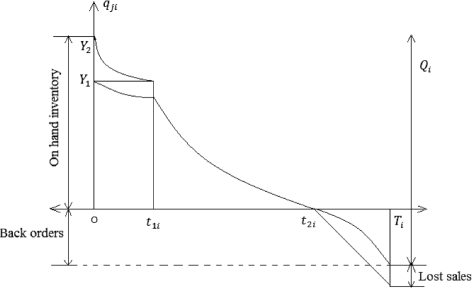Fig. 8

Case-I: When shortages do not occur;

The differential equation describing the inventory level q 1i (t)(i=1,2,...,n) for the ith item in [0,t 1i ] is given by

$$\frac{dq_{1i}(t)}{dt}=-D_{i}-\varepsilon_{1i}q_{1i}(t) ~~~ 0\leq t \leq t_{1i}$$
(14)

with boundary condition q 1i (t 1i )=0.

Using the boundary conditions, we have the solution of Eq. (14) as

$$q_{1i}(t)=\frac{a_{i}}{\varepsilon_{1i}+b_{i}}\left[ e^{(\varepsilon_{1i}+b_{i})(t_{1i}-t)}-1\right] ~~~0\leq t \leq t_{1i}$$
(15)

And inventory level q 2i (t)(i=1,2,...,n) governed by the differential equations, for the ith item in [0,t 1i ]

$$\frac{dq_{2i}(t)}{dt}=-\varepsilon_{2i}q_{2i}(t) ~~~ 0\leq t \leq t_{1i}$$
(16)

with initial conditions q 2i (0)=w i . Using the initial conditions, we have the solution Eq. (16) as

$$q_{2i}(t)=w_{i}e^{-\varepsilon_{2i}t} ~~~ 0\leq t \leq t_{1i}$$
(17)

During the time t[t 1i ,t 2i ], the inventory in OY 1 is reduced due to the joint effects of demand and deterioration. Hence, the inventory level q 2i (t)(i=1,2,...,n) at OY 1 is governed by the differential equations as

$$\frac{dq_{2i}(t)}{dt}=-D_{i}-\varepsilon_{2i}q_{2i}(t) ~~~ t_{1i}< t \leq t_{2i}$$
(18)

with boundary conditions q 2i (t 2i )=0.

The solution of Eq. (18) is given by

$$q_{2i}(t)=\frac{a_{i}}{\varepsilon_{2i}+b_{i}}\left[ e^{(t_{2i}-t)(b_{i}+\varepsilon_{2i})}-1\right] ~~~t_{1i}< t \leq t_{2i}$$
(19)

At the point t=t 1i , by the continuity property of q 2i (t), we have from Eqs. (17) and (19) as

\begin{aligned} w_{i}e^{-\varepsilon_{2i}t_{1i}}&=\frac{a_{i}}{\varepsilon_{2i}+b_{i}}\left[ e^{(t_{2i}-t_{1i})(b_{i}+\varepsilon_{2i})}-1\right]\\ \Rightarrow t_{2i}&=t_{1i}+\frac{1}{b_{i}+\varepsilon_{2i}}\ln \left[1+\frac{w_{i}}{a_{i}}(b_{i}+\varepsilon_{2i})e^{-\varepsilon_{2i}t_{1i}}\right] \end{aligned}
(20)

Here t 2i is a function of t 1i . We take the derivative of t 2i with respect to t 1i on the both sides of Eq. (20) as

$$\frac{dt_{2i}}{dt_{1i}}=\frac{1+\frac{w_{i}b_{i}}{a_{i}}e^{-\varepsilon_{2i}t_{1i}}}{\left(1+\frac{w_{i}b_{i}}{a_{i}}e^{-\varepsilon_{2i}t_{1i}}\right)+\frac{w_{i}\varepsilon_{2i}}{a_{i}}e^{-\varepsilon_{2i}t_{1i}}}<1.$$
(21)

Then $$\frac {dt_{2i}}{dt_{1i}}-1<0$$ holds.

Case-II: When shortage occurs;

If q 3i (t) be the inventory level at time t over the time period [t 2i ,T i ], then the governing differential equations are

$$\frac{dq_{3i}(t)}{dt}=-D_{i}\delta_{i} ~~~ t_{2i}< t \leq T_{i}$$
(22)

with the boundary conditions q 3i (t 2i )=0

Using the boundary conditions, we have the solution of Eq. (22) as

$$q_{3i}(t)=a_{i}\delta_{i}(t_{2i}-t)$$
(23)

The ordering quantity over the replenishment cycle for the ith item is

$$\begin{array}{@{}rcl@{}} Q_{i}&=&q_{1i}(0)+q_{2i}(0)-q_{3i}(0) \\ &=& \frac{a_{i}}{\varepsilon_{1i}+b_{i}}\left[e^{(\varepsilon_{1i}+b_{i})t_{1i}}-1\right]+w_{i}+(-a_{i}\delta_{i}(T_{i}-t_{2i}))\\ &=&\frac{a_{i}}{\varepsilon_{1i}+b_{i}}\left[e^{(\varepsilon_{1i}+b_{i})t_{1i}}-1\right]+w_{i}+a_{i}\delta_{i}t_{3i} \end{array}$$

where t 3i =T i t 2i .

The maximum inventory level in each cycle for ith item is

$$\begin{array}{@{}rcl@{}} M_{i}&=&q_{1i}(0)+q_{2i}(0)\\ &=& \frac{a_{i}}{\varepsilon_{1i}+b_{i}}\left[e^{(\varepsilon_{1i}+b_{i})t_{1i}}-1\right]+w_{i} \end{array}$$

Now we calculate the different types of cost for ith item (i=1,2,...,n), which are based on previous equations.

The ordering cost in each cycle for ith item is A i

Holding cost in each cycle for ith item in OY 1 is

$$\begin{array}{@{}rcl@{}} &=&\tilde{c_{1i}}\left(\int_{0}^{t_{1i}}q_{1i}(t)dt+\int_{t_{1i}}^{t{2i}}q_{2i}(t)dt\right)\\ &=&\tilde{c_{1i}}\left\lbrace \int_{0}^{t_{1i}}w_{i}e^{-\varepsilon_{2i}t}dt+\int_{t_{1i}}^{t_{2i}}\frac{a_{i}}{\varepsilon_{2i}+b_{i}}\left[e^{(b_{i}+\varepsilon_{2i})(t_{2i}-t)}-1\right]dt\right\rbrace \\ &=&\tilde{c_{1i}}\left\lbrace\frac{w_{i}}{\varepsilon_{2i}}\left(1-e^{-\varepsilon_{2i}t_{1i}}\right)+\frac{a_{i}}{(b_{i}+\varepsilon_{2i})^{2}}\left[e^{(b_{i}+\varepsilon_{2i})(t_{2i}-t_{1i})}-(t_{1i}-t_{2i})(b_{i}+\varepsilon_{2i})-1\right]\right\rbrace \end{array}$$

Holding cost in each cycle for ith item in Y 1 Y 2 is

$$\begin{array}{@{}rcl@{}} &=&\tilde{c_{2i}}\int_{0}^{t_{1i}}q_{1i}(t)dt\\ &=&\tilde{c_{2i}}\int_{0}^{t_{1i}}\frac{a_{i}}{\varepsilon_{1i}+b_{i}}\left(e^{(\varepsilon_{1i}+b_{i})(t_{1i}-t)}-1 \right)dt\\ &=&\frac{\tilde{c_{2i}}a_{i}}{(\varepsilon_{1i}+b_{i})^{2}}\left(e^{(\varepsilon_{1i}+b_{i})t_{1i}}-t_{1i}(\varepsilon_{1i}+b_{i})-1\right) \end{array}$$

Sales revenue cost in each cycle for ith item is

$$\begin{array}{@{}rcl@{}} &=&\tilde{s_{i}}\left\lbrace\int_{0}^{t_{2i}}D_{i}dt+\int_{0}^{T_{i}}D_{i}\delta_{i}dt\right\rbrace\\ &=&\tilde{s_{i}}\left\lbrace\int_{0}^{t_{1i}}D_{i}dt+\int_{t_{1i}}^{t_{2i}}D_{i}dt+ \int_{0}^{T_{i}}D_{i}\delta_{i}dt\right\rbrace\\ &=&\tilde{s_{i}}\left\lbrace\int_{0}^{t_{1i}}\left(a_{i}+b_{i}q_{1i}(t)\right)dt+\int_{t_{1i}}^{t_{2i}}\left(a_{i}+b_{i}q_{2i}(t)\right)dt+\int_{t_{1i}}^{T_{i}}a_{i}\delta_{i}dt\right\rbrace\\ &=&\tilde{s_{i}}\left[a_{i}t_{2i}+\frac{a_{i}b_{i}}{(\varepsilon_{1i}+b_{i})^{2}}\left\lbrace e^{(\varepsilon_{1i}+b_{i})t_{1i}}-(\varepsilon_{1i}+b_{i})t_{1i}-1\right\rbrace \right.\\ & & \left.\,\qquad \quad+\frac{a_{i}b_{i}}{(\varepsilon_{2i}+b_{i})^{2}}\left\lbrace e^{(b_{i}+\varepsilon_{2i})(t_{2i}-t_{1i})}-(t_{2i}-t_{1i})(b_{i}+\varepsilon_{2i})-1\right\rbrace\right] \end{array}$$

Opportunity cost due to lost sales per cycle for ith item is

$$\begin{array}{@{}rcl@{}} &=&\tilde{R_{i}}\int_{t_{2i}}^{T_{i}}D_{i}\left(1-\delta_{i}\right)dt\\ &=&\tilde{R_{i}}a_{i}(1-\delta_{i})t_{3i} \end{array}$$

Purchase cost in each cycle for ith item is

$$\begin{array}{@{}rcl@{}} &=&\tilde{c_{pi}}Q_{i}\\ &=&\tilde{c_{pi}}\left\lbrace\frac{a_{i}}{\varepsilon_{1i}+b_{i}}\left(e^{(\varepsilon_{1i}+b_{i})t_{1i}}-1\right)+w_{i}-a_{i}\delta_{i}t_{3i}\right\rbrace \end{array}$$

Shortage cost in each cycle for ith item is

$$\begin{array}{@{}rcl@{}} &=&\tilde{c_{3i}}\int_{t_{2i}}^{T_{i}}-q_{3i}(t)dt\\ &=&\tilde{c_{3i}}\int_{t_{2i}}^{T_{i}}a_{i}\delta_{i}(t-t_{2i})dt\\ &=&\frac{1}{2}\tilde{c_{3i}}a_{i}\delta_{i}t_{3i}^{2} \end{array}$$

Total average profit per unit time of our model is

$$\begin{array}{@{}rcl@{}} {Average\ profit} &=& {sales\ revenue - holding\ cost - ordering\ cost - shortage\ cost} \\ &&{- opportunity\ cost - purchasing\ cost} \end{array}$$
$$\begin{array}{@{}rcl@{}} {} \tilde{PT}(t_{2},t_{3})\!&\!=&\sum\limits_{i=1}^{n}\frac{1}{t_{2i}+t_{3i}} \left[ \tilde{s_{i}} \left(a_{i}t_{2i}+\frac{a_{i}b_{i}}{(\varepsilon_{1i}+b_{i})^{2}}\left\lbrace e^{(\varepsilon_{1i}+b_{i})t_{1i}}-(\varepsilon_{1i}+b_{i})t_{1i}-1\right\rbrace \right.\right.\\ & & \left. +\frac{a_{i}b_{i}}{(\varepsilon_{2i}+b_{i})^{2}}\left\lbrace e^{(b_{i}+\varepsilon_{2i})(t_{2i}-t_{1i})}-(t_{2i}-t_{1i})(b_{i}+\varepsilon_{2i})-1\right\rbrace +a_{i}\delta_{i}t_{2i}\right)-\tilde{A_{i}} \\ & & \,-\,\tilde{c_{1i}}\left\lbrace\frac{w_{i}}{\varepsilon_{2i}} \left(1-e^{-\varepsilon_{2i}t_{1i}}\right)\,+\,\frac{a_{i}}{(b_{i}+\varepsilon_{2i})^{2}}\left(e^{(b_{i}+\varepsilon_{2i})(t_{2i}-t_{1i})}\,-\,(t_{2i}\,-\,t_{1i})(b_{i}\,+\,\varepsilon_{2i})\,-\,1\right)\right\rbrace \\ & & -\frac{\tilde{c_{2i}}a_{i}}{(\varepsilon_{1i}+b_{i})^{2}}\left\lbrace e^{(\varepsilon_{1i}+b_{i})t_{1i}}-t_{1i}(\varepsilon_{1i}+b_{i})-1\right\rbrace - \frac{1}{2}\tilde{c_{3i}}a_{i}\delta_{i}t_{3i}^{2}-\tilde{R_{i}}a_{i}(1-\delta_{i})t_{3i} \\ & & \left. \!-\tilde{c_{pi}}\left\lbrace\frac{a_{i}}{\varepsilon_{1i}+b_{i}}\left(e^{(\varepsilon_{1i}+b_{i})t_{1i}}-1\right)+w_{i}+a_{i}\delta_{i}t_{3i}\right\rbrace \right] \end{array}$$
(24)

where t 2=(t 21,t 22,...,t 2n )T and t 3=(t 31,t 32,...,t 3n )T.

Our problem is to maximize the total average profit under two constraints, budget constraints and space constraints. Hence, the multi-item fuzzy inventory model is given by

\begin{aligned} Z=\text{Maximize} & \tilde{PT}(t_{2},t_{3}) \\ \text{subject to} & \sum\limits_{i=1}^{n}\tilde{c_{3i}}Q_{i} \leq \tilde{F};\\ & \sum\limits_{i=1}^{n}\tilde{c_{pi}}Q_{i} \leq \tilde{B};\\ & t_{2}\geq 0,\ t_{3}\geq 0 \end{aligned}
(25)

where t 2=(t 21,t 22,.....,t 2n )T and t 3=(t 31,t 32,.....,t 3n )T are decisions variables and $$Q_{i}=\frac {a_{i}}{\varepsilon _{1i}+b_{i}}\left [e^{(\varepsilon _{1i}+b_{i})t_{1i}}-1\right ]+w_{i}+a_{i}\delta _{i}t_{3i}$$.

### Solution Methodology

To solved this multi-item fuzzy inventory model with two constraints, space constraints and budget constraints, apply the expected value ETFN technique in the proposed model (Eq. 25) and transform in to the crisp model, then the current model can be written as follows:

\begin{aligned} Z^{*}=\text{Maximize} & E[\tilde{PT}(t_{2},t_{3})]\\ \text{subject to} & \sum\limits_{i=1}^{n}E[{\tilde{c_{3i}}}Q_{i}] \leq E[\tilde{F}];\\ & \sum\limits_{i=1}^{n}E[\tilde{c_{pi}}Q_{i}] \leq E[\tilde{B}];\\ & t_{2}\geq 0,\ t_{3}\geq 0 \end{aligned}
(26)

where t 2=(t 21,t 22,.....,t 2n )T and t 3=(t 31,t 32,.....,t 3n )T are decision variables.

## Numerical Illustration

To illustrate the proposed multi-item two-warehouse inventory model, we have considered an inventory problem with purchasing cost, shortage cost, available shortage space, selling price, capacity of owned warehouse, and holding cost. In most inventory problems in real life, we observed that the different costs and other parameters are normally vague and imprecise in nature. For example, the holding cost of an item is supposed to be dependent on the amount of storage. Similarly, the total shortage cost depends upon the amount of stock in a scheduling period, etc. So, in this inventory system, the total average profit in a scheduling period may be uncertain, and uncertainties may be associated with these variables, and the above goals and parameters are normally vague and increase. This uncertain and vague nature of a parameter can be capture by linear or non-linear fuzzy numbers.

Sometimes, the use of linear fuzzy number in describing decision satisfaction may lead to loss of information. Moreover, the linear fuzzy number does not allow decision makers to provide any kind of biasness towards objective. In order to capture decision maker biasness, we may used non-linear fuzzy number to represent the inventory model in a much more realistic way. In developing countries like India and Bangladesh, different cost, related to inventory model, rapidly changes from time to time. To capture those rapid changes, we have chosen appropriate non-linear fuzzy numbers, for example exponential fuzzy numbers may be more suitable than other fuzzy numbers. In this paper, we have considered holding cost, shortage cost, purchasing cost, opportunity cost, etc. as exponential fuzzy numbers. Nowadays, due to fluctuation of the market, purchasing cost increases rapidly. This purchasing cost rapidly increases for some items (like onion, rice, and potato). To capture both property uncertainness and fleetness for some items, we have considered the multi-item two-warehouse inventory model under exponential fuzzy environment. Therefore, for this proposed inventory problem, the holding cost, purchasing cost, shortage cost, available shortage space, capacity of owned warehouse, and selling price are considered in the exponential fuzzy environment. The exponential fuzzy parameters of the proposed inventory model are given in Table 1, and its input crisp parameters are given in Table 2.

Our problem is to find out the length of the inventory (T i ), optimal order quantity(Q i ), and total average profit (Z ) for the proposed inventory problem. The optimal solutions of the proposed inventory problem with respect to different deterioration are presented in Table 3.

Using the parameters given in Tables 1 and 2, problem (26) have been solved by using a soft computing technique, generalized reduced gradient (GRG) method. We have also considered problem (26) for non-deteriorating items and solve it using the parameters of Tables 1 and 2 and the results are given in Table 3.

## Sensitivity Analysis

Sensitivity analysis are performed for maximization of the total average profit (Z ) in inventory problem, length of the inventory (T i ), and order quantity (Q i ) with respect to change of deteriorations (ε ki )(k=1,2). From Table 3, the following decisions can be constructed, which are also reflected in Figs. 9, 10, 11, and 12.

1. (i)

If the value of deterioration rate ε ki (k=1,2) increases, then the total average profit Z decreases (cf. Figs. 9 and 10).

2. (ii)

Table 3 shows that length of the inventory per cycle T i decreases with increase of ε 1i (cf. Fig. 10)

3. (iii)

Again Table 3 shows that the order quantity per cycle Q i increases when the deterioration rate increases (cf. Fig. 12).Fig. 9Fig. 10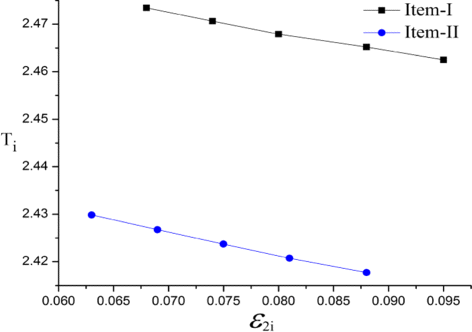Fig. 11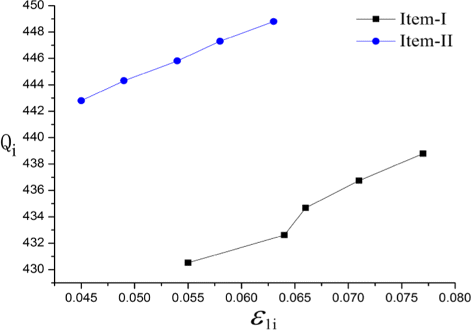Fig. 12

## Conclusion

For the first time, possibility, necessity, and credibility measures of exponential trapezoidal fuzzy numbers and its expected value are presented here. In Example 1, we have shown the advantage of the expected value operator technique for exponential trapezoidal fuzzy numbers. The multi-item deterministic inventory model is developed for deteriorating items with stock-dependent demand, permitting shortage and finite warehouse capacity. In this model we have considered different deterioration costs and holding costs for OY 1 and Y 1 Y 2 because of different conservation conditions. Finally, this model is also formulated under exponential trapezoidal fuzzy environment and solved using a soft computing technique, generalized reduced gradient method. The model is also discussed for non-deteriorating items as a special case of the deteriorating items and results are given in Table 3. To show the validity of the proposed model, few sensitivity analyses with respect to the different rates of deterioration have been carried out. The proposed method can also be applied for multi-objective, multi-constraint, and multi-supplier inventory problem with non-linear demand, which may be areas of future work.

## References

1. 1

Zadeh, AL: Fuzzy sets. Inf. Comput. 8, 338–353 (1965).

2. 2

Zadeh, AL: Fuzzy sets as a basis for a theory of possibility. Fuzzy Sets Syst. 1, 3–28 (1978).

3. 3

Dubois, D, Prade, H: Possibility theory. Plenum press, New York (1988).

4. 4

Klir, JG: On fuzzy set interpretation of possibility theory. Fuzzy Sets Syst. 108, 263–273 (1992).

5. 5

Yager, RR: On the specificity of a possibility distribution. Fuzzy Sets Syst. 50, 279–292 (1992).

6. 6

Liu, B, Liu, KY: Expected value of fuzzy variable and fuzzy expected value models. IEEE Trans. Fuzzy Syst. 10, 445–450 (2002).

7. 7

Dubois, D, Prade, H: The mean value of a fuzzy number. Fuzzy Sets Syst. 24, 279–300 (1987).

8. 8

Carlsson, C, Fuller, R: On possibilistic mean and variance of fuzzy number. Fuzzy Sets Syst. 122, 315–326 (2001).

9. 9

Heilpern, S: The expected value of a fuzzy number. Fuzzy Sets Syst. 47, 81–86 (1992).

10. 10

Ghare, MP, Schrader, PG: A model for exponential decaying inventory. J. Ind. Eng. 14, 238–243 (1963).

11. 11

Das, K, Roy, KT, Maiti, M: Multi-item inventory model with quantity-dependent inventory costs and demand-dependent unit cost under imprecise objective and restrictions: a geometric programming approach. Prod. Plan. Control. 11, 781–788 (2000).

12. 12

Maiti, KM, Maiti, M: Production policy for damageable items with variable cost function in a imperfect production process via genetic algorithm. Math. Comput. Model. 42, 977–990 (2005).

13. 13

Mondal, S, Maiti, M: Multi-item fuzzy inventory EOQ models using genetic algorithm. Comput. Ind. Eng. 44, 105–117 (2002).

14. 14

Mousavi, MS, Hajipour, V, Niaki, ATS, Alikar, N: Optimizing multi-item multi period inventory control system with discounted cash flow and inflation: Two calibrated meta-heuristic algorithms. Appl. Math. Model. 37, 2241–2256 (2013).

15. 15

Pasandideh, RHS, Niaki, ATS, Sharareh, S: Optimizing a bi-objective multi-product EPQ model with defective item, rework and limited orders: NSGA-II and MOPSO algorithms. J. Manuf. Syst. 32, 764–770 (2013).

16. 16

Wee, MH, Lo, CC, Hsu, HP: A multi-objective joint replenishment inventory model of deteriorated items in a fuzzy environment. Eur. J. Oper. Res. 197, 620–631 (2009).

17. 17

Lau, H, Lau, A: The multi-product multi-constraint newsboy problem: Application, formulation and solution. J. Oper. Manag. 13, 153–162 (1995).

18. 18

Nahmias, S, Schmidt, PC: An efficient heuristic for the multi-item newsboy problem with a single constraint. Nav. Res. Logist. Q. 31, 463–474 (1997).

19. 19

Vairaktarakis, GL: Robust multi-item newsboy models with a budget constraint. Int. J. Prod. Econ. 66, 213–226 (2000).

20. 20

Misra, BR: Optimum production lot-size model for a system with deteriorating inventory. Int. J. Prod. Res. 13, 495–505 (1975).

21. 21

Raafat, F: Survey of literature on continuously deteriorating inventory model. J. Oper. Res. Soc. 42, 27–37 (1991).

22. 22

Goyel, KS, Giri, CB: Recent trends in modeling of deteriorating inventory. Eur. J. Oper. Res. 134, 1–16 (2001).

23. 23

Benkherouf, AL: Deterministic order level inventory model for deteriorating items with two storage facilities. Int. J. Prod. Econ. 48, 167–175 (1997).

24. 24

Cohen, AM: Joint pricing and ordering policy for exponentially decaying inventory with known demand. Nav. Res. Logist. Q. 24, 2520–268 (1977).

25. 25

Kang, S, Kim, I: A study on the price and production level of the deteriorating inventory system. Int. J. Prod. Res. 21, 449–460 (1983).

26. 26

Goyal, KS, Gunasekaran, A: An integrated production inventory marketing model for deteriorating item. Comput. Ind. Eng. 28, 755–762 (1995).

27. 27

Sarma, VK: A deterministic inventory model with two level of storage and an optimum release rule. Opsearch. 20, 175–180 (1983).

28. 28

Murdeshwar, AT, Sathe, SY: Some aspects of lot size model with two levels of shortage. Opsearch. 22, 255–262 (1985).

29. 29

Pakkala, MPT, Achary, KK: A deterministic inventory model for deteriorating items with two warehouses and finite replenishment rate. Eur. J. Oper. Res. 57, 71–76 (1992).

30. 30

Bhunia, KA, Maiti, M: A two-warehouse inventory model for deteriorating items with a linear trend in demand and shortages. J. Oper. Res. Soc. 49, 287–292 (1998).

31. 31

Goswami, A, Chuaudhuri, SK: On an inventory model with two levels of storage and stock-dependent demand rate. Int. J. Syst. Sci. 29, 249–254 (1998).

32. 32

Zhou, WY: A multi-warehouse inventory model for items with time varying demand and shortages. Comput. Oper. Res. 30, 2115–2134 (2003).

33. 33

Maity, K, Maiti, M: Possibility and necessity constraints and their defuzzification-A multi-item production-inventory scenario via optimal control theory. Eur. J. Oper. Res. 177, 882–896 (2007).

34. 34

Yao, SJ, Le, MH: Economic production quantity quantity for fuzzy demand quantity and fuzzy production quantity. Eur. J. Oper. Res. 109, 203–211 (1998).

35. 35

Nia, RA, Far, HM, Niaki, ATS: A fuzzy vendor managed inventory of multi-item economic order quantity model under shortage: An ant colony optimization algorithm. Int. J. Prod. Econ. 155, 259–271 (2014).

36. 36

Kar, S, Bhunia, M, Maiti, M: A fuzzy deteriorating multi-items EOQ model with price dependent demand under budgetary constraint. AMSE, France. 19, 45–59 (2000).

37. 37

Roy, KT, Maiti, M: A multi-item displayed EOQ model in fuzzy environment. J. Fuzzy Math. 8, 881–888 (2000).

38. 38

Kumar, A, Shing, P, Kaur, A: Ranking of generalized exponential fuzzy numbers using integral value approach. Int. J. Adv. Soft Comput. Appl. 2, 1–10 (2010).

39. 39

Xu, J, Zhou, X: A class of multi-objective expected value decision-making model with birandom coefficients and its application to flow shop scheduling problem. Inf. Sci. 179, 2997–3017 (2009).

Download references

### Competing Interests

The authors declare that they have no competing interests.

## Author information

Authors

### Contributions

All authors contributed to the research equally. All authors read and approved the final manuscript.

### Corresponding author

Correspondence to Dipankar Chakraborty.

## Ethics declarations

### Publisher’s Note

Springer Nature remains neutral with regard to jurisdictional claims in published maps and institutional affiliations.

## Rights and permissions

Open Access This article is distributed under the terms of the Creative Commons Attribution 4.0 International License(http://creativecommons.org/licenses/by/4.0/), which permits unrestricted use, distribution, and reproduction in any medium, provided you give appropriate credit to the original author(s) and the source, provide a link to the Creative Commons license, and indicate if changes were made.

Reprints and Permissions